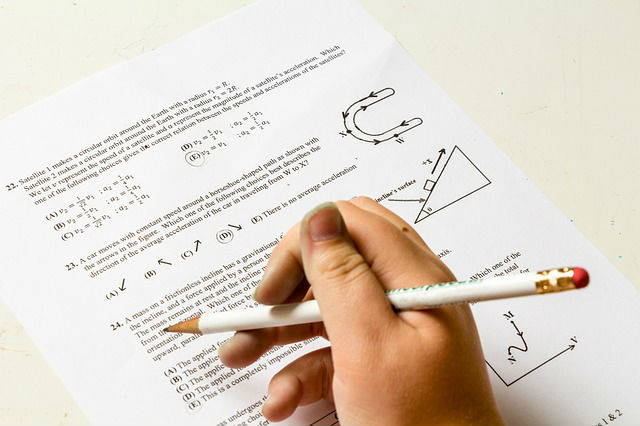# Solution of Physics Numericals for class 9 (set 1 Q13)

Last updated on November 6th, 2020 at 09:13 am

## Problem Statement

A cricket ball of mass 100 g moving at a speed of 30 m/s is brought to rest by a player in 0.03 s. find the average force applied by the player.

Solution:
mass =m= 100 g = 100/1000 kg = 0.1 kg

initial velocity = u=30 m/s

Final velocity = v=0

Time duration = t = 0.03 s

Acceleration = (v-u)/t = (0-30)/0.03 = – 3000/3 = -1000 m/s^2

Average Force = mass * acceleration = 0.1 * (-1000) N = -100N

The magnitude of force is 100 N and the negative sign signifies that it is applied in the direction opposite to the direction of motion of the ball.